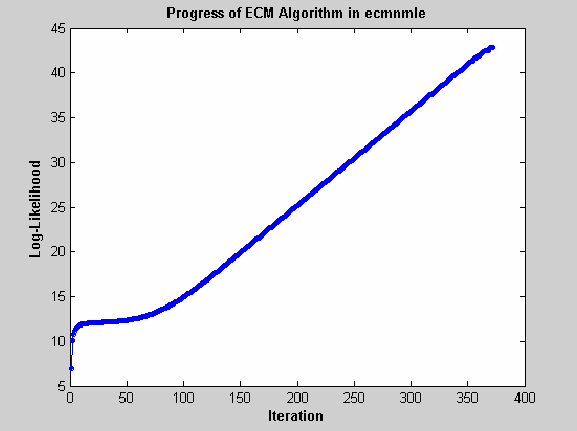## Troubleshooting Multivariate Normal Regression

This section provides a few pointers to handle various technical and operational difficulties that might occur.

### Biased Estimates

If samples are ignored, the number of samples used in the estimation is less than `NumSamples`. Clearly the actual number of samples used must be sufficient to obtain estimates. In addition, although the model parameters `Parameters` (or mean estimates `Mean`) are unbiased maximum likelihood estimates, the residual covariance estimate `Covariance` is biased. To convert to an unbiased covariance estimate, multiply `Covariance` by

`$\text{Count}/\left(\text{Count}-1\right),$`

where `Count` is the actual number of samples used in the estimation with `Count` ≤ `NumSamples`. None of the regression functions perform this adjustment.

### Requirements

The regression functions, particularly the estimation functions, have several requirements. First, they must have consistent values for `NumSamples`, `NumSeries`, and `NumParams`. As a rule, the multivariate normal regression functions require

and the least-squares regression functions require

`$\text{Count}×\text{NumSeries}\le \text{NumParams},$`

where `Count` is the actual number of samples used in the estimation with

`$\text{Count}\le \text{NumSamples}\text{.}$`

Second, they must have enough nonmissing values to converge. Third, they must have a nondegenerate covariance matrix.

Although some necessary and sufficient conditions can be found in the references, general conditions for existence and uniqueness of solutions in the missing-data case, do not exist. Nonconvergence is usually due to an ill-conditioned covariance matrix estimate, which is discussed in greater detail in Nonconvergence.

### Slow Convergence

Since worst-case convergence of the ECM algorithm is linear, it is possible to execute hundreds and even thousands of iterations before termination of the algorithm. If you are estimating with the ECM algorithm regularly with regular updates, you can use prior estimates as initial guesses for the next period's estimation. This approach often speeds up things since the default initialization in the regression functions sets the initial parameters b to zero and the initial covariance C to be the identity matrix.

Other improvised approaches are possible although most approaches are problem-dependent. In particular, for mean and covariance estimation, the estimation function `ecmnmle` uses a function `ecmninit` to obtain an initial estimate.

### Nonrandom Residuals

Simultaneous estimates for parameters b and covariances C require C to be positive-definite. So, the general multivariate normal regression routines require nondegenerate residual errors. If you are faced with a model that has exact results, the least-squares routine `ecmlsrmle` still works, although it provides a least-squares estimate with a singular residual covariance matrix. The other regression functions fail.

### Nonconvergence

Although the regression functions are robust and work for most “typical” cases, they can fail to converge. The main failure mode is an ill-conditioned covariance matrix, where failures are either soft or hard. A soft failure wanders endlessly toward a nearly singular covariance matrix and can be spotted if the algorithm fails to converge after about 100 iterations. If `MaxIterations` is increased to 500 and display mode is initiated (with no output arguments), a typical soft failure looks like this.This case, which is based on 20 observations of five assets with 30% of data missing, shows that the log-likelihood goes linearly to infinity as the likelihood function goes to 0. In this case, the function converges but the covariance matrix is effectively singular with a smallest eigenvalue on the order of machine precision (`eps`).

For the function `ecmnmle`, a hard error looks like this:

```> In ecmninit at 60 In ecmnmle at 140 ??? Error using ==> ecmnmle Full covariance not positive-definite in iteration 218. ```

From a practical standpoint, if in doubt, test your residual covariance matrix from the regression routines to ensure that it is positive-definite. This is important because a soft error has a matrix that appears to be positive-definite but actually has a near-zero-valued eigenvalue to within machine precision. To do this with a covariance estimate `Covariance`, use `cond(Covariance)`, where any value greater than `1/eps` should be considered suspect.

If either type of failure occurs, however, note that the regression routine is indicating that something is probably wrong with the data. (Even with no missing data, two time series that are proportional to one another produce a singular covariance matrix.)